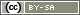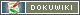This translation is older than the original page and might be outdated. See what has changed.

This is an old revision of the document!

# Combined Questions

You might want to ask different options about one item. For example the rating of a characteristic and its importance. This is how to implement this.

Example 1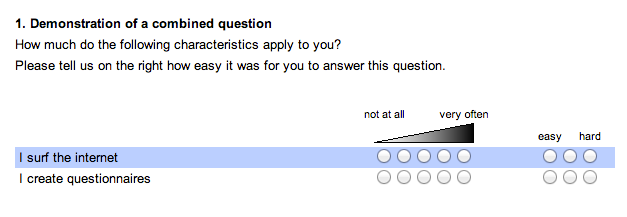Example 2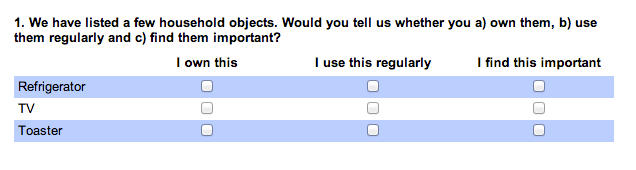## Function

Combined questions are a combination of different single questions from the “list of questions”. The title and the description as well as the item texts originate from the first question. So does the leftmost input option. However, the items of the first question were compressed a little so there was more space for further input options. Those originate from other questions.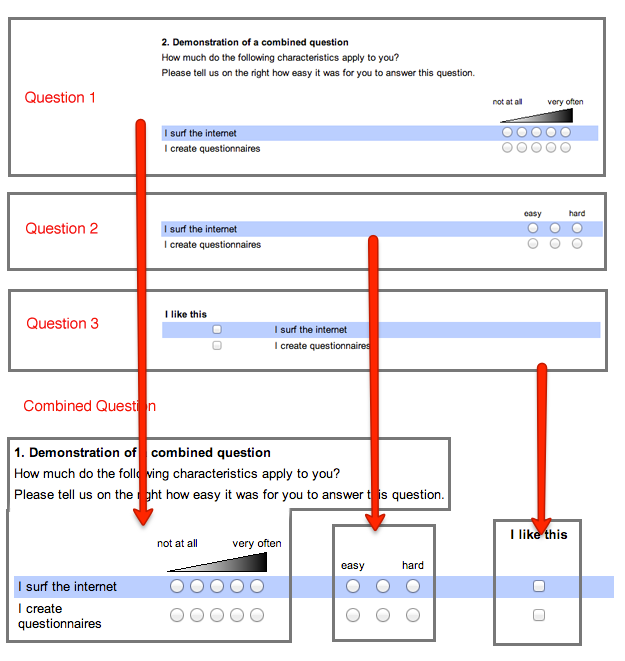The instruction focusses at first on example one (two questions), then on example two (three questions)

Attention: Not all question types are suitable for combined questions. For example, a dropdown selection does not use an own line for all options (which corresponds to an item). Thus this type cannot be combined with other questions. To combine those see (Placing input fields anywhere).

## Step by step

1. Create the leftmost part of the question: As question type choose the one that should show its input fields on the left. In example one this would be a 5 point “scale - extremes labeled”.
2. Create all items resp. input options for this question.
3. Duplicate the question. (on the upper left of your screen: Duplicate question).
4. The 'List of questions' now lists this question twice: The original and a copy. The questions are basically the same, they just have different IDs.
5. The copy – the “second” question – should now be marked as such. For example, add a “(secondary)” to the Term.
6. Change the question type and the verbal anchor to what you would like them to be on the right of your combined question. In example one this would be a 3-point-scale with different labels and without visual anchorage.
7. In Compose Questionnaire mode, open your questionnaire at the point your combined question should appear.
8. Place the flexible element PHP-Code into the page and use the command `question()` to show question one. You may also drag and drop the question into the PHP-code-field.
9. Next to the ID of question one (e.g. “AB01”), add the option “combine=” and the ID of question two (e.g. “combine=AB02”). You may also add other options for the question.
`question('AB01','combine=AB02');  // Combines AB01 with AB02`

Hint: When combined with another question, regardless of their ID, the items of question 2 will use the same position (not IDs!) as the items of question one. For example, if the items of question one have the IDs 3, 4 and 5 but the position 1, 2 and 4, question two will ask for the items with the position 1, 2 and 4, regardless of their ID.

It might happen, that the item AB01_03 corresponds to the item AB02_01, because the position of the items were changed. Consider this when evaluating the results. You can avoid confusion if you don't change any item position after duplicating the question. If needed anyways, you can change the item order of the output by using the function `question()`.

Hint: Randomizing the item order is no problem. Be aware, the setting of question one is the only one regarded, while the other questions follow the first so there are no inconsistencies.

Hint: Questions kan be dragged-and-dropped into the questionnaire-page or be included by using PHP. If you do both on one page, the question will be shown twice and you will get the respective error messages.

## Combining more than two questionsTo combine more than two questions, follow the steps above. At step nine, use the option combine several times for every question to be added. The labels above the columns (in example two: “I use this regularly”) are entered as Text on top of the options in the “Edit question” page.

PHP-Code

```question(
'T201', 'combine=T202','combine=T203',
);```

To have sufficient space for the labels, you may need to adjust the widths of the colums. Enter a value (in this example 150 pixels) at Width of input column under the Alignment of input boxes tab and set the Alignment of the input fields to “center”.

## Display options

By default, the input fields of the questions have a gap of 16 pixels. By using the option gap you may adjust this behavior. The setting applies to the whole combined question. Different spaces between individual question parts are not possible at present.

• gap=none
No gap between input fields
• gap=<n>
A gap of n pixels between input fields
• gap=line
A line is displayed between input fields

The labels of the questions other than the first are not displayed by default. If you want to display the item texts, add the option combine_items.

Hint: If a multiple choice or selection question are set to show the labels on the right (“Position of the input fields - left hand”), they are always displayed, regardless of the option at combine_items.

• combine_items=yes
Show item texts for a question (needs to be specified for all questions)

PHP-Code

```// No spce between input fields
question('AB01','combine=AB02','gap=none');
question('AB01','combine=AB02','gap=0');
// Line between input fields
question('AB01','combine=AB02','gap=line');
// Space of 20 pixels beween input fields
// and item labels for the second (!) question only
question(
'AB01','gap=20','combine_items=no'
'combine=AB02', 'combine_items=yes'
);```